# GSEB Solutions Class 12 Maths Chapter 12 Linear Programming Ex 12.1

Gujarat Board GSEB Textbook Solutions Class 12 Maths Chapter 12 Linear Programming Ex 12.1 Textbook Questions and Answers.

## Gujarat Board Textbook Solutions Class 12 Maths Chapter 12 Linear Programming Ex 12.1

Solve the following Linear Programming Problems graphically:

Question 1.
Maximise Z = 3x + 4y
subject to constraints: x + y ≤ 4, x ≥ 0, y ≥ 0.
Solution:
Objective function is
Z = 3x + 4y
Constraints are
x + y ≤ 4, x ≥ 0, y ≥ 0

(i) consider the line
x + y = 4.
It passes through the points (4, 0) and (0, 4).
Putting x = 0, y = 0 in x + y ≤ 4, we get 0 ≤ 4 which is true.
Therefore, origin lies in the region x + y ≤ 4.
x ≥ 0 is the region to the right of y-axis and y ≥ 0 is the region on and above x-axis.
∴ The feasible region is ∆ OAB.
At (4, 0), Z = 3x + 4y = 3 × 4 + 0 = 12
At (0, 4), Z = 3x + 4y = 0 + 4 × 4 = 16
At (0, 0), Z = 0.
∴ Maximum value of Z = 16.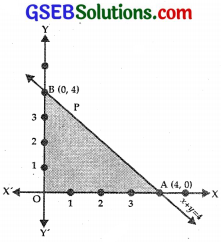Question 2.
Minimise Z = – 3x + 4y
subject to x + 2y ≤ 8, 3x + 2y ≤ 12, x ≥ 0, y ≥ 0.
Solution:
Objective function Z = – 3x + 4y
Constraints are x + 2y ≤ 8, 3x + 2y ≤ 12, x ≥ 0, y ≥ 0

(i) Consider the line
x + 2y = 8
It passes through A(8, 0) and B(0, 4).
Putting x = 0, y = 0 in
x + 2y ≤ 8, 0 ≤ 8 which is true.
⇒ region x + 2y ≤ 8 lies on and below AB.

(ii) The line 3x + 2y = 12 passes through P(4, 0), Q(0, 6).
Putting x = 0, y = 0 in 3x + 2y ≤ 12.
⇒ 0 ≤ 12, which is true.
∴ Region 3x + 2y ≤ 12 lies on and below PQ.

(iii) x ≥ 0, the region lies on and to the right of y-axis.

(iv) y ≥ 0 lies on and above x-axis.

(v) Solving the equations x + 2y = 8 and 3x + 2y = 12,
we get x = 2, y = 3
⇒ R is (2,3), where AB and PQ intersect.
The shaded region OPRB is the feasible region.
At P(4, 0), Z = – 3x + 4y = – 12 + 0 = – 12
At R(2, 3), Z = – 6 + 12 = 6
At B(0, 4), Z = 0 + 16 = 16
At Q(0, 0), Z = 0
Thus, minimum value of Z is – 12 at P(4, 0) [Neglecting the value at (0, 0)].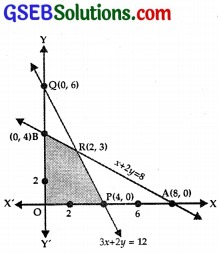Question 3.
Maximise Z = 5x + 3y.
subject to 3x + 5y ≤ 15, 5x + 2y ≤ 10, x ≥ 0, y ≥ 0.
Solution:
The objective function is Z = 5x + 3y.
Constraints are Y
3x + 5y ≤ 15,
5x + 2y ≤ 10, x ≥ 0, y ≥ 0

(i) Consider the line 3x + 5y = 15, which passes through A(5, 0) and B(0, 3).
Putting x = 0, y = 0, in 3x + 5y ≤ 15
⇒ 0 ≤ 15 which is true.
∴ Region 3x + 5y ≤ 15 lies on and below AB.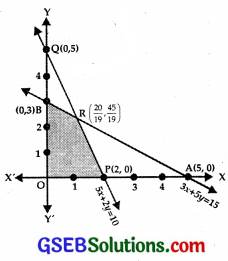(ii) The line 5x + 2y = 10 passes through P(2, 0) and Q(0, 5).
Put x = 0, y = 0 in 5x + 2y ≤ 10
∴ 0 ≤ 10, which is true.
Region 5x + 2y ≤ 10 lies on and below PQ.

(iii) x ≥ 0 region lies on and to the right of y-axis.

(iv) y ≥ 0 lies on and above x-axis.

(v) The feasible region is the shaded area OPRB.
Solving the equations, 3x + 5y – 15 and 5x + 2y = 10,
we get x = $$\frac{20}{19}$$, y = $$\frac{45}{19}$$.
⇒ AB and PQ intersect at R ($$\frac{20}{19}$$, $$\frac{45}{19}$$).
At P(2, 0), Z = 5x + 3y = 10 + 0 = 10
At R($$\frac{20}{19}$$, $$\frac{45}{19}$$),
Z = $$\frac{100}{19}$$ + $$\frac{135}{19}$$ = $$\frac{235}{19}$$
At B(0, 3), Z = 9
At O(0, 0), Z = 0
∴ Maximum value of Z = $$\frac{235}{19}$$ at ($$\frac{20}{19}$$, $$\frac{45}{19}$$).Question 4.
Minimise P = 3x + 5y
subject to x + 3y ≥ 3, x + y ≥ 2, x, y ≥ 0.
Solution:
The objective function is Z = 3x + 5y.
Constraints are x + 3y ≥ 3, x + y ≥ 2, x, y ≥ 0.

(i) consider the line
x + 3y = 3.
This passes through A(3, 0) and B(0, 1).
Putting x = 0, y = 0 in x + 3y > 3
⇒ 0 ≥ 3, which is not true.
Region x + 3y ≥ 3 lies on and above AB.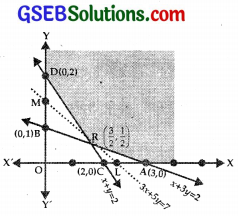(ii) The line x + y = 2 passes through C(2, 0) and D(0, 2)
Putting x = 0, y = 0 in x + y ≥ 2
⇒ 0 ≥ 2, which is not true.
⇒ Region x + y ≥ 2 lies on and above the line CD.

(iii) x ≥ 0, this region is on and to the right of y-axis.

(iv) y ≥ 0 is on and above the x-axis.

(v) The lines x + 3y = 3 and x + y = 2 intersect at R($$\frac{3}{2}$$, $$\frac{1}{2}$$).

(vi) The feasible region is XARDY.
At A(3, 0), Z = 3x + 5y = 9 + 0 = 9.
At R($$\frac{3}{2}$$, $$\frac{1}{2}$$), Z = $$\frac{9}{2}$$ + $$\frac{5}{2}$$ = $$\frac{14}{2}$$ = 7.
At D(0, 2), Z = 0 + 5 × 2 = 10.
∴ Minimum value of Z at R($$\frac{3}{2}$$, $$\frac{1}{2}$$) is 7.
The feasible region XARDY is unbounded. Consider the inequality 3x + 5y < 7
The line 3x + 5y = 7 passes through ($$\frac{7}{3}$$, 0) and (0, $$\frac{7}{5}$$).
3x + 5y < 7 has no common point with feasible region
∴ Minimum value of Z = 7 at R($$\frac{3}{2}$$, $$\frac{1}{2}$$).Question 5.
Maximise Z = 3x + 2y
subject to x + 2y ≥ 10, 3x + y ≤ 15, x, y ≥ 0.
Solution:
The objective function is Z = 3x + 2 y.
Constraints are x + 2y ≤ 10,
3x + y ≤ 15,
x, y ≥ 0

(i) Consider the line
x + 2 y = 10.
This line passes through A(10, 0) and B(0, 5).
Putting x = 0, y = 0 in x + 2y ≤ 10
0 ≤ 10, which is true.
⇒ x + 2y ≤ 10 lies on and below AB.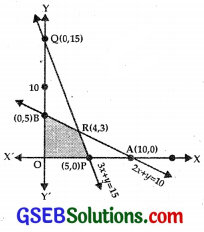(ii) The line 3x + y = 15 passes through P(5, 0) and Q(0, 15).
Putting x = 0, y = 0 is 3x + y ≤ 15, we get 0 ≤ 15, which is true.
⇒ 3x + y ≤ 15 lies on and below PQ.

(iii) x ≥ 0 lies on and to the right of y-axis.

(iv) y ≥ 0 lies on and above x-axis.

(v) The feasible region is OPRB.

(vi) Equations are
x + 2 y = 10 …………….. (1)
3x + y = 15 ………………… (2)
Multiplying (2) by 2 to obtains
6x + 2y – 30 ……………. (3)
Subtracting (1) from (3),
5x – 20
⇒ x = 4
From (2), y = 15 – 3x = 15 -12 = 3.
Lines (1) and (2) meet at R(4, 3).
Z = 3x + 2y
Now, At P(5, 0) Z = 15 + 0 = 15
At R(4, 3) Z = 12 + 6 = 18
At B(0, 5) Z = 0 + 10 = 10
∴ Maximum value of Z = 18 at R(4, 3).Question 6.
Minimise Z = x + 2y
subject to 2x + y ≥ 3, x + 2y ≥ 6, x, y ≥ 0.
Show that minimum of Z occurs at more than two points.
Solution:
Objective function Z = x + 2y.
Constraints are 2x + y ≥ 3, x + 2y ≥ 6, x, y ≥ 0.

(i) Consider the line
2x + y = 3.
This passes through A($$\frac{3}{2}$$, 0) and B(0, 3).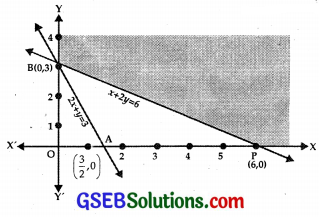Putting x = 0, y = 0 in 2x + y ≥ 3. we get 0 ≥ 3, which is not true.
∴ The region 2x + y ≥ 3 is on and above AB.

(ii) The line x + 2y = 6 passes through P(6, 0) and B(0, 3).
Putting x = 0, y = 0 in x + 2y ≥ 6
⇒ 0 ≥ 6 which is not true.
∴ x + 2y ≥ 6 region lies on PB and above it.

(iii) x ≥ 0 lies on and to the right of y-axis.

(iv) y ≥ 0 lies on and above x-axis.
Shaded area XPBY is the feasible region.
At P(6, 0), Z = x + 2y = 6 + 0 = 6.
At B(0, 3), Z = 0 = 6 = 6
⇒ At all points on PB, Z = 6
Moreover x + 2y < 6 lies below PB which has no common point in feasible region.
Thus, the minimum value of Z is 6.Question 7.
Minimise and Maximise Z = 5x + 10y
subject to x + 2y ≤ 120, x + y ≥ 60, x – 2y ≥ 0, x, y ≥ 0.
Solution:
The objective functions is
Z = 5x + 10y.
Constraints are
x + 2y ≤ 120,
x + y ≥ 60,
x – 2y ≥ 0,
x, y ≥ 0

(i) The line x + 2y = 120 passes through A(120, 0) and 5(0, 60)
Putting x = 0, y = 0 is x + 2y ≤ 120,
we get 0 ≤ 120, which is true.
⇒ x + 2y ≤ 120 lies on AB and below AB.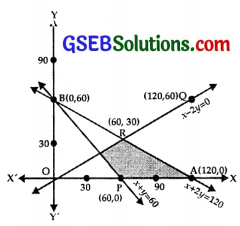(ii) The line x + y = 60 passes through P(60, 0) and B(0, 60).
Putting x = 0 and y = 0 in x + y ≥ 60, which is not true.
⇒ x + y ≥ 60 lies on PB or above it.

(iii) The line x – 2y = 0 passes through O(0, 0) and Q(120, 60).
Putting x = 60, y = 0 in x – 2y ≥ 0, 60 ≥ 0, which is true.
⇒ x – 2y = 0 region is on QQ and below it.

(iv) x ≥ 0 lies on y-axis on its right.

(v) y ≥ 0 lies on x-axis and above it.
⇒ Feasible region is PARS which has been shaded.Question 8.
Minimise and Maximise Z = x + 2y
subject to x + 2y ≥ 100, 2x – y ≤ 0, 2x + y ≤ 200, x, y ≥ 0.
Solution:
Objective functions is Z = x + 2y.
Constraints are
x + 2y ≥ 100,
2x – y ≤ 0,
2x + y ≤ 200,
x, y ≥ 0

(i) The line x + 2y – 100, passes through A(100, 0) and B(0, 50).
Putting x = 0, y = 0 in x + y ≥ 100 or 0 ≥ 100, which is not true.
∴ Region x + 2y ≥ 100 lies on and above AB.

(ii) The line 2x – y = 0 passes through O(0, 0) and C(50, 100).
The point (0, 50) lies in the region 2x – y ≤ 0
∴ Region 2x – y ≤ 0 lies on and above OC.

(iii) The line 2x + y = 200 passes through A(100, 0) and D(0, 200).
Put x = 0, y = 0 in 2x + y ≤ 200, i.e., 0 ≤ 200, which is true.
⇒ 2x + y ≤ 200 lies on AD and below it.

(iv) x ≥ 0 lies on y-axis and on the right of it.

(v) y ≥ 0 lies on and above x-axis.
Feasible region is the shaded area BECD.
The point E is the intersection of
OC : 2x – y = 0 and AB : x + 2y = 100
i.e., The point E is (20, 40).
The point C(50, 100) lies on 2x + y = 200 and 2x – y = 0.
Now,
At B(0,50), Z = x + 2y = 100
At E(20, 40), Z = 20 + 80 = 100
At C(50, 100), Z = 50 + 200 = 250
At D(0, 200), Z = 0 + 2 × 200 = 400
Minimum value of Z = 100 at all points on the line segment joining the points B(0, 50) and E(20, 40).
Maximum value of Z = 400 at D(0, 200).Question 9.
Maximise Z = – x + 2y, subject to constraints:
x ≥ 3, x + y ≥ 5, x + 2y ≥ 6, y ≥ 0.
Solution:
The objective function is Z = – x + 2y. The constraints are:
x ≥ 3, x + y ≥ 5, x + 2y ≥ 6, y ≥ 0

(i) The line x + y = 5 passes through A(5, 0), B(0, 5).
Putting x = 0, y = 0 in x + y ≥ 5, we get 0 ≥ 5, which is not true.
⇒ x + y ≥ 5 lies on and above AB.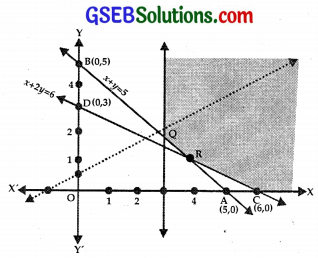(ii) The line x + 2y = 6 passes through C(6, 0), D(0, 3).
Putting x = 0, y = 0 in x + 2y ≥ 6,
we get 0 ≥ 6, which is not true.
⇒ x + 2y ≥ 6 lies on and above CD.

(iii) x ≥ 3 lies on PQ or on the right of it.

(iv) y ≥ 0 lies on and above x-axis.

(v) The feasible region is PQRCX.

(a) Solving x = 3 and x + y = 5, we get x = 3, y = 2.
∴ These lines meet at Q(3, 2).

(b) x + y = 5 and x + 2y = 6 meet at R,
i.e., the point R is (4, 1).
At Q(3, 2), Z = – x + 2y = – 3 + 4 = 1
At R(4, 1), Z = – 4 + 2 = – 2
At C(6, 0), Z = – 6 + 0 = – 6
The maximum value of Z is 1 but the feasible region is unbounded.
Consider the inequality – x + 2y > 1
The line – x + 2y = 1 passes through (- 1, 0) and (0, $$\frac{1}{2}$$).
Putting x = 0, y = 0 in – x + 2y > 1, we get 0 > 1, which is not true.
⇒ – x + 2y > 1 lies above the line – x + 2y = 1.
∴ Feasible region of – x + 2y > 1 have many points in common.
Therefore, there is no maximum value.Question 10.
Maximise Z = x + y subject to
x – y ≤ – 1, – x + y ≤ 0, x, y ≥ 0.
Solution:
Objective function is Z = x + y.
Constraints are x – y ≤ – 1,
– x + y ≤ 0, x, y ≥ 0.

(i) The line x – y = – 1 passes through (- 1, 0) and (0, 1).
Putting x = 0, y = 0 in x – y ≤ – 1,
we get 0 ≤ – 1 which is not true.
⇒ x – y ≤ – 1 lies on and above
AB : x – y = – 1.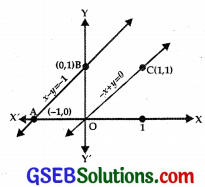(ii) the line – x + y = 0 passes through O(0, 0) and C(1, 1).
Putting x = 0, y = 1 in – x + y ≤ 0,
we get 1 ≤ 0, which is not true.
⇒ – x + y ≤ 0 lies on and below OC.

(iii) x ≥ 0 lies on and on the right of y-axis.

(iv) y ≥ 0 lies on and above x-axis.
There is no common region, i.e., their is no feasible region.
⇒ There is no maximum value of Z.